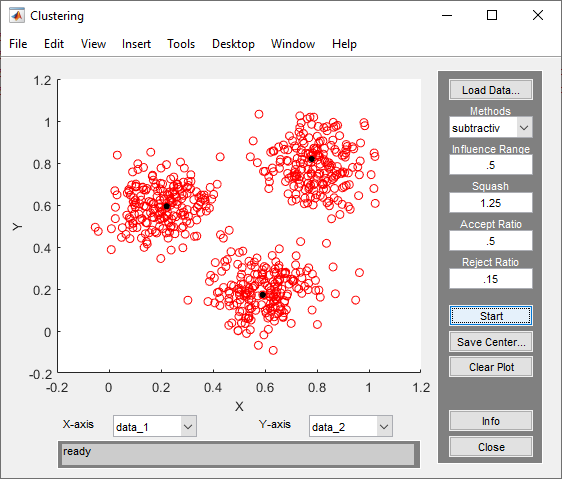Main Content

## Data Clustering Using Clustering Tool

The Clustering tool implements the fuzzy data clustering functions `fcm` and `subclust`, and lets you perform clustering on data. For more information on the clustering methods, see Fuzzy Clustering.

To open the tool, at the MATLAB® command line, type:

`findcluster`Use the Clustering tool to perform the following tasks:

1. Load and plot the data.

2. Perform the clustering.

3. Save the cluster center.

Access the online help topics by clicking or using the Help menu.

### Load and Plot Data

To load a data set, perform either of the following actions:

• Click , and select the file containing the data.

• Open the Clustering Tool with a data set directly by calling `findcluster` with the data set as an input argument.

For example, enter:

`findcluster('clusterdemo.dat')`

The data set file must have the extension `.dat`. Each line of the data set file contains one data point. For example, if you have 5-dimensional data with 100 data points, the file contains 100 lines, and each line contains five values.

The Clustering tool works on multidimensional data sets, but displays only two of those dimensions on the plot. To select other dimensions in the data set for plotting, you can use the drop-down lists under X-axis and Y-axis.

### Cluster Data

To start clustering the data:

1. Choose the clustering function `fcm` (fuzzy C-Means clustering) or `subtractiv` (subtractive clustering) from the drop-down menu under Methods.

2. Set options for:

• Fuzzy c-means clustering using the Cluster Num, Max Iteration, Min, and Exponent fields. For information on these options, see `fcm`.

• Subtractive clustering using the Influence Range, Squash, Aspect Ratio, and Reject Ratio fields. For information on these options, see `subclust`.

3. Cluster the data by clicking .

Once the clustering is complete, the cluster centers appear in black as shown in the next figure.Tip

Using the Clustering tool, you can obtain only the computed cluster centers. To obtain additional information for:

• Fuzzy c-means clustering, such as the fuzzy partition matrix, cluster the data using `fcm`.

• Subtractive clustering, such as the range of influence in each data dimension, cluster the data using `subclust`.

To use the same clustering data with either `fcm` or `subclust`, first load the data file into the MATLAB workspace. For example, at the MATLAB command line, type:

`load clusterdemo.dat`

### Save Cluster Centers

To save the cluster centers, click .

Download now